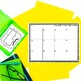# Area and Perimeter of Rectilinear Figures Task CardsSubject
Resource Type
File Type

PDF

(827 KB|27 pages)
Product Rating
3.8
(9 Ratings)
Standards
Also included in:
1. Practice and master the Area and Perimeter standards with this Area & Perimeter Task Cards Bundle. 10 different sets of task cards are included (5 different products) to cover all of the area and perimeter standards. From tiling and unit squares to rectangles to rectilinear figures, this task
\$17.00
\$13.00
Save \$4.00
• Product Description
• StandardsNEW

Build upon students' knowledge of area and perimeter of rectangles with these Area and Perimeter of Rectilinear Figures Task Cards. Standards expect students to not only identify the area and perimeter of a rectangle, but also of rectilinear figures. These Area & Perimeter Task cards contain 2 sets of open-ended task cards that can be used to practice either area OR perimeter of rectangles so that you can use them to practice both skills. They intentionally do not have directions on the cards and the recording sheet. This allows you to have students practice area, perimeter, or both. With two sets, you can have one set be used for perimeter, and the other used for practicing area.

DISTRIBUTIVE PROPERTY- This product also demonstrates the distributive property as students decompose the shape into smaller parts and then add them together (area as additive). You may also be interested in my Distributive Property task cards to practice this skill without the area model.

WHAT'S INCLUDED:
• 2 sets of 36 task cards (one with unit squares and one without)

• Recording sheet

This product includes 2 sets of task cards (each set has 36 cards in it) as well as a recording sheet with a mustache theme. Students first work to identify the area and perimeter of a rectilinear figure when given unit squares. The second set has students finding the area and/or the perimeter when given measurements.

With 36 cards, these are perfect for beginning with a whole class guided practice before students continue working independently or in small groups. These task cards are perfect for Scoot and Solve the Rooms as students practice area and perimeter of rectangles.

Other Area & Perimeter Products:

Elving Around: An Area & Perimeter Unit

CLICK HERE to follow me and be notified of future products as soon as they are posted.

_______________________________________________________________

You may not redistribute, edit, sell, or otherwise post this product on the internet. You may, however, post a link for otherstopurchase themselves.

Recognize area as additive. Find areas of rectilinear figures by decomposing them into non-overlapping rectangles and adding the areas of the non-overlapping parts, applying this technique to solve real world problems.
Use tiling to show in a concrete case that the area of a rectangle with whole-number side lengths 𝘢 and 𝘣 + 𝘤 is the sum of 𝘢 × 𝘣 and 𝘢 × 𝘤. Use area models to represent the distributive property in mathematical reasoning.
Apply the area and perimeter formulas for rectangles in real world and mathematical problems. For example, find the width of a rectangular room given the area of the flooring and the length, by viewing the area formula as a multiplication equation with an unknown factor.
Solve real world and mathematical problems involving perimeters of polygons, including finding the perimeter given the side lengths, finding an unknown side length, and exhibiting rectangles with the same perimeter and different areas or with the same area and different perimeters.
Multiply side lengths to find areas of rectangles with whole-number side lengths in the context of solving real world and mathematical problems, and represent whole-number products as rectangular areas in mathematical reasoning.
Total Pages
27 pages
Included
Teaching Duration
N/A
Report this Resource to TpT
Reported resources will be reviewed by our team. Report this resource to let us know if this resource violates TpT’s content guidelines.